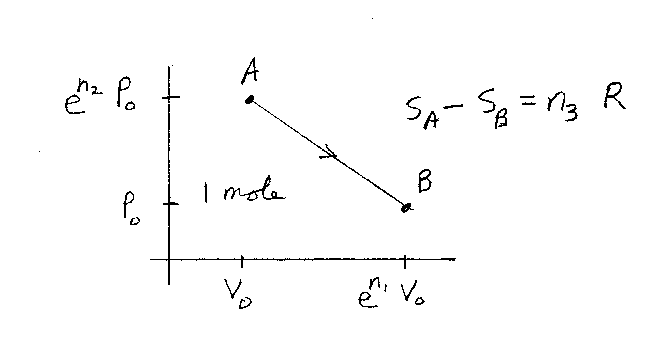Problem D12: Consider one mole of an ideal gases in a container. The gas starts off in the state A, which has a volume V0 and a pressure en2P0. The gas ends up in the state B, which has a volume of en1V0 and a pressure P0. The gas undergoes the reversible process as shown in the figure. What is the change in entropy of the gas. If the change in entropy is SA-SB = n3R, what is n3? Note that n1, n2, and n3 are unitless. R is the gas constant.n1 = n2 = Input n3:

If you are currently in my class, you can record your grade by entering your name and student ID number (without the leading zeros) below and clicking on "record grade".
 First Name = Last Name = ID = Problem: